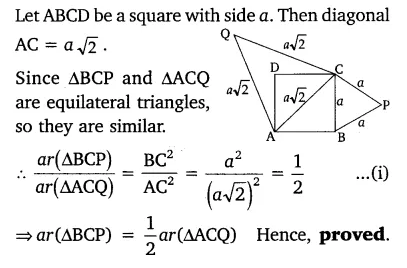# Prove that the area of an equilateral triangle described on one side of a square

Prove that the area of an equilateral triangle described on one side of a square is equal to half the area of the equilateral triangle described on one of its diagonals.